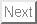Proceeding of International Conference
Quentin Brandon, Tetsushi Ueta, Daniele Fournier-Prunaret, Method of Bifurcation Analysis of Hybrid Nonlinear Systems, Proc. NOMA'07, 59-62, Toulouse, Dec. 2007.
Abstract: Systems showing continuous and discrete dimensions, in other words hybrid systems, are present in many domains such as mechanical (apparition of frictions) or electronic systems (switching component). The solution function usually remains continuous but presents points of non-derivability where discrete changes occur. Some of the most widely studied applications are usually related to electrical engineering, such as power converters investigated as piecewise smooth systems by di Bernardo , Tse  or Banerjee ; or some PLL models as introduced By Acco , refering to this type of model as ``hybrid sequential.'' Little work has been done in the bifurcation analysis of nonlinear hybrid models, apart from Kawakami, Ueta and Kousaka . As for hybrid linear models, they can be analysed using rigorous analytical methods, so exact solutions are obtained and used for analyses of the total dynamical behavior, as did Kabe . But the nonlinear characteristics of many systems make this option possible only for approximations. Instead, we prefer using numerical methods. We propose using a Poincar´e map in order to obtain a discrete linearized approximation and conduct the bifurcation analysis using conventional methods. We consider the analysis results of the Alpazur oscillator, which reveals an unsual bifurcation structure: the interactions between the equilibrium point at some state and the corresponding switching condition make appear a fractal bifurcation structure, with an infinite number of bifurcation curves focusing towards a limit set.